## How to solve indefinite integral of sine 2x divided by square sine of x plus 3 by dx ?

Short answer: indefinite integral of sine 2x divided by square sine of x plus 3 by dx is ln|(sin^2)x+3|+C. ∫ ((sin 2x)/(sin^2 x +3))dx is not particulary hard integral. You can solve it in 8 easy steps. We will walk you through and explain everything. Let's start.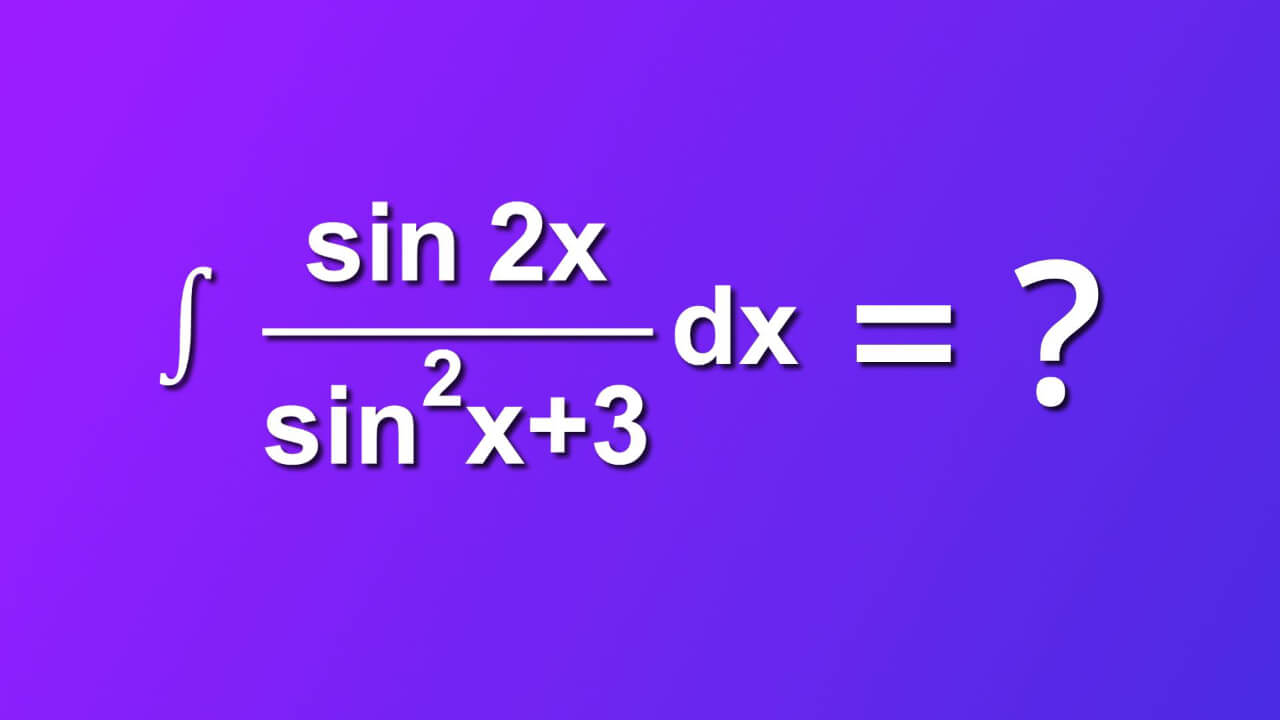## Required assumtions

Usually, we have some additional info about function f of (x). In our case:

1. f(x) belongs to real numbers
2. f(x) is integrable in that domain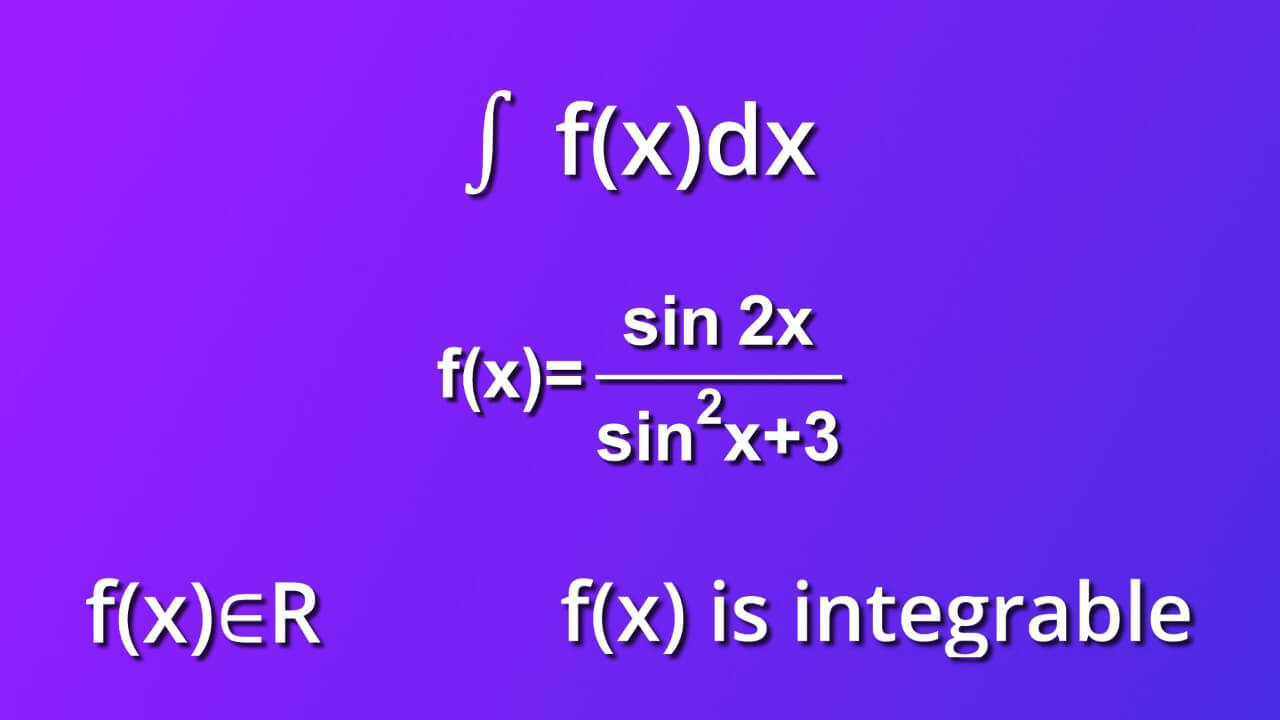## Step by step solution of ∫ ((sin 2x)/(sin^2 x +3))dx

We will solve ∫ ((sin 2x)/(sin^2 x +3))dx in 8 easy steps. Let's get started

### Step 1

From symbol dx we know that differential of variable x indicates that the variable of integration is x. In that case, to solve this integral we may apply so called u−substitution method. We do it in order to modify the integral in the way that enable using some of formulas for finding integrals. But first let’s get advantage of formula sine 2s equals 2 sine s multiplied by cosine s. In our case s is x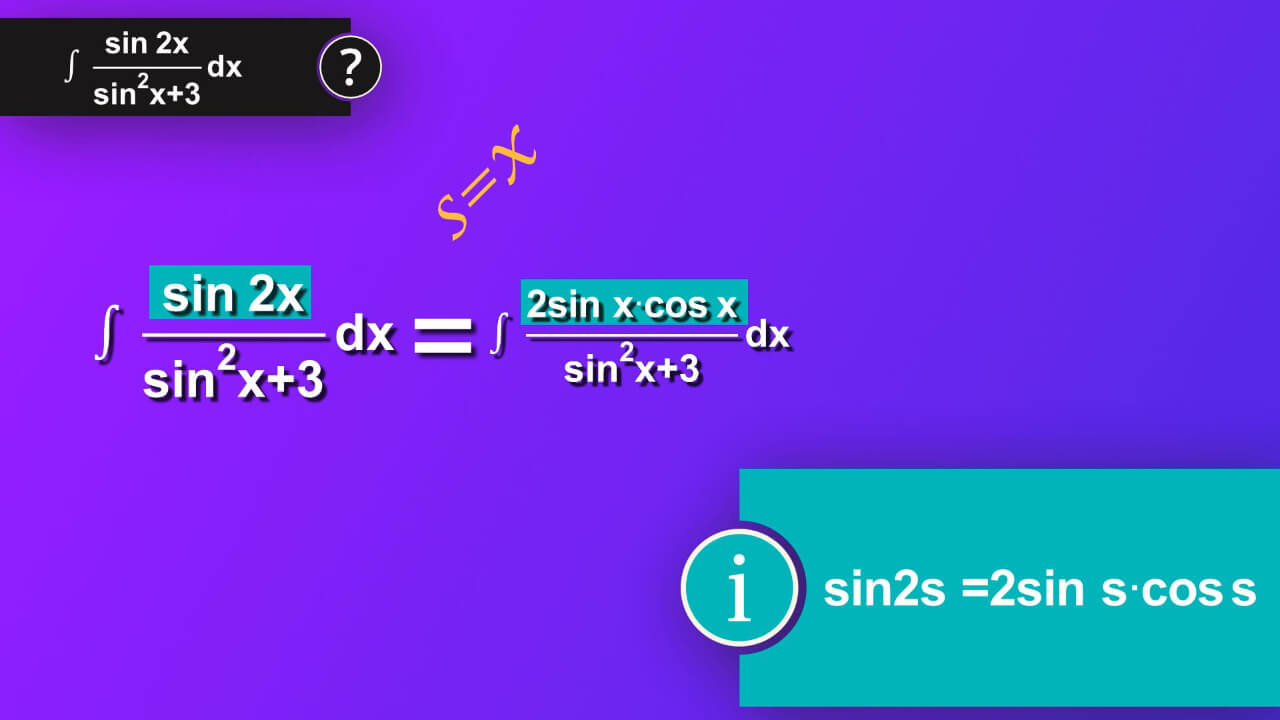### Step 2

and we can rewrite our numerator as 2 sine x cosine x. Now, we try to substitute expression sine square x plus 3. We say u is equal sine square x plus 3. We also have to know what the derivative of u is. So we write derivative of u equals d (sine square x plus 3). So we’ve got du is equal to derivative of sine square x plus 3 multiplied by dx. From rules that we can apply to derivatives, we know that derivative of sine square x plus 3 equals derivative of sine square x plus derivative of 3 and the whole expression is multiplied by dx. Let’s focus for a moment on derivative of sine square x. We can distinguish here two functions – sinus and rising to the power of 2. Thus, we can apply formula which says derivative of function g which is a function of h of variable s equals derivative of function g as a function of h of s multiplied by derivative of internal function h of s. In our case g is responsible for rising to the power of 2 and h is sinus s, where s is x.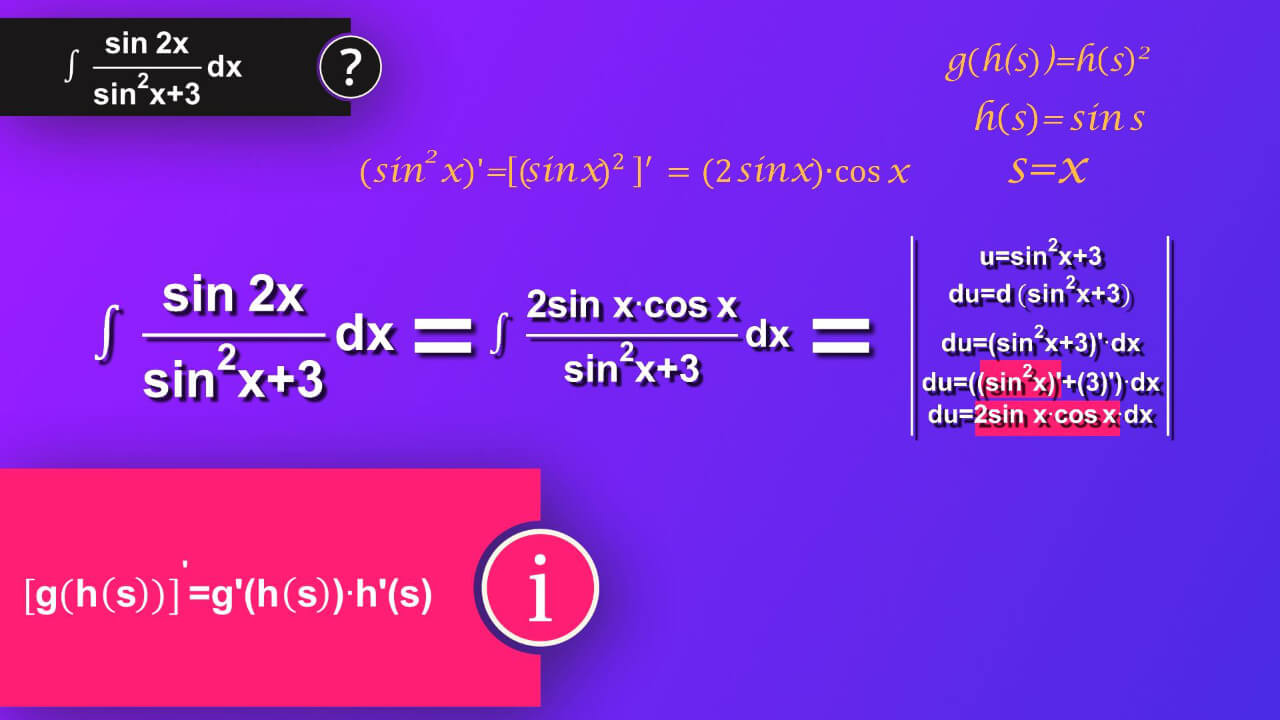### Step 3

In result, derivative of sine x rise to second power is equal to 2 sine x as derivative of s rise to n equals n multiplied by s rise to power n minus 1. In our case s is x and n is 2. Then, we have to multiply it by derivative of internal function and in our case it is derivative of sin x. Knowing that derivative of sine s equals cosine s and s is x in our case, we can write second part of our derivative solution as cosine x. We also now that derivative of constant equals zero, and in our case derivative of 3 is zero. Finally, du is equal to 2 sine of x cosine x multiplied by dx. We can leave the expression like this as in a moment we insert it in exactly that form to our main equation.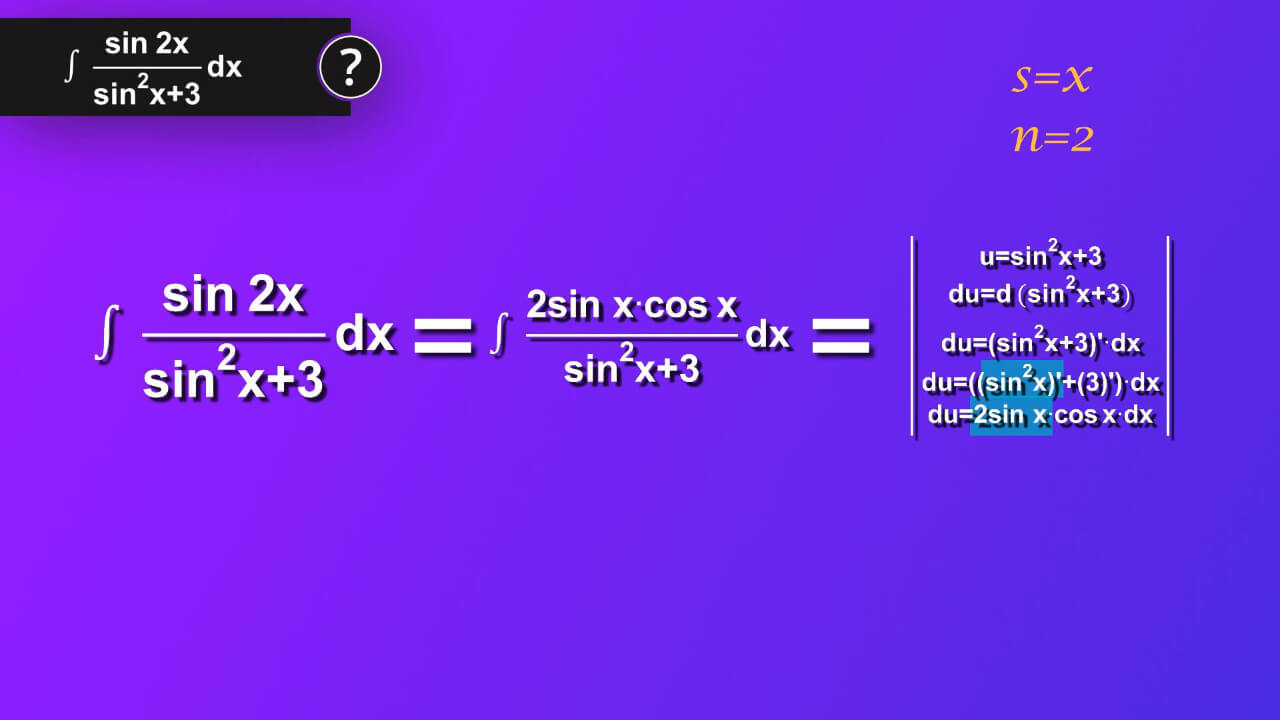### Step 4

Let’s make use of our u. We insert u instead of square sine of x plus 3 to our main equation and instead of the whole numerator multiplied by dx we can now insert du. Thus, we have integral of du divided by u in our main equation.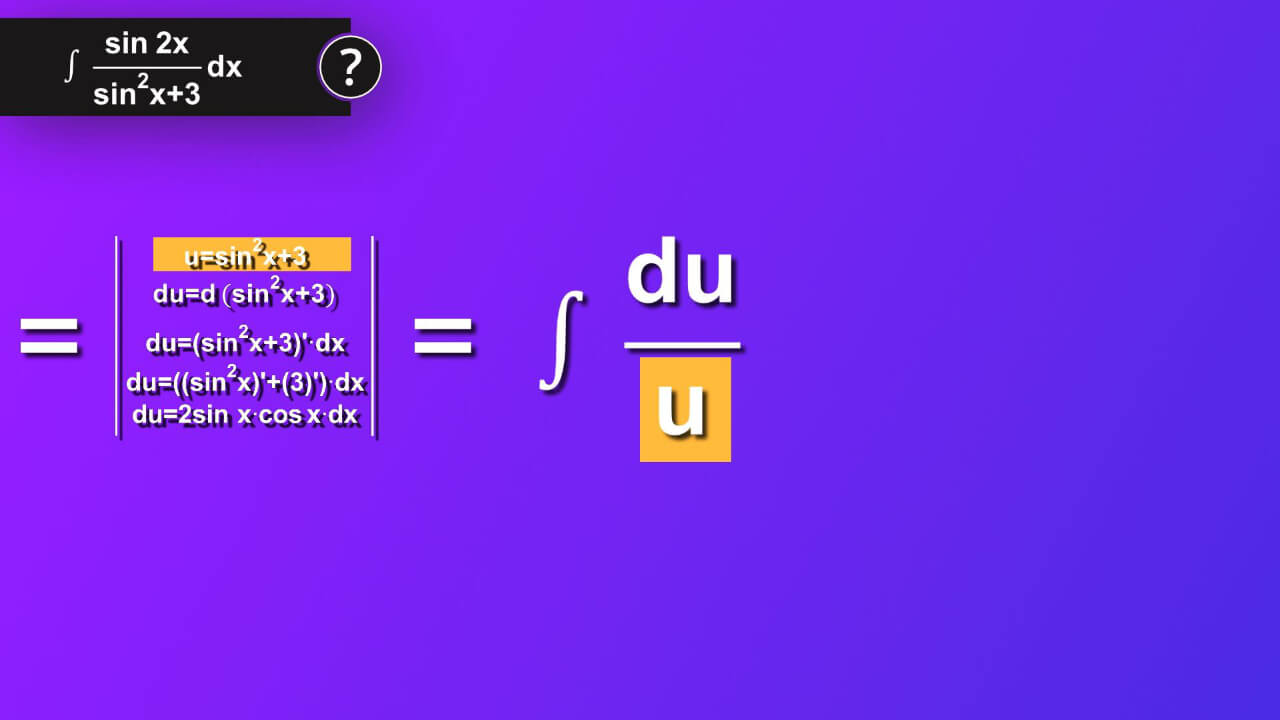### Step 5

We can rewrite this expression as integral of one divided by u multiplied by du.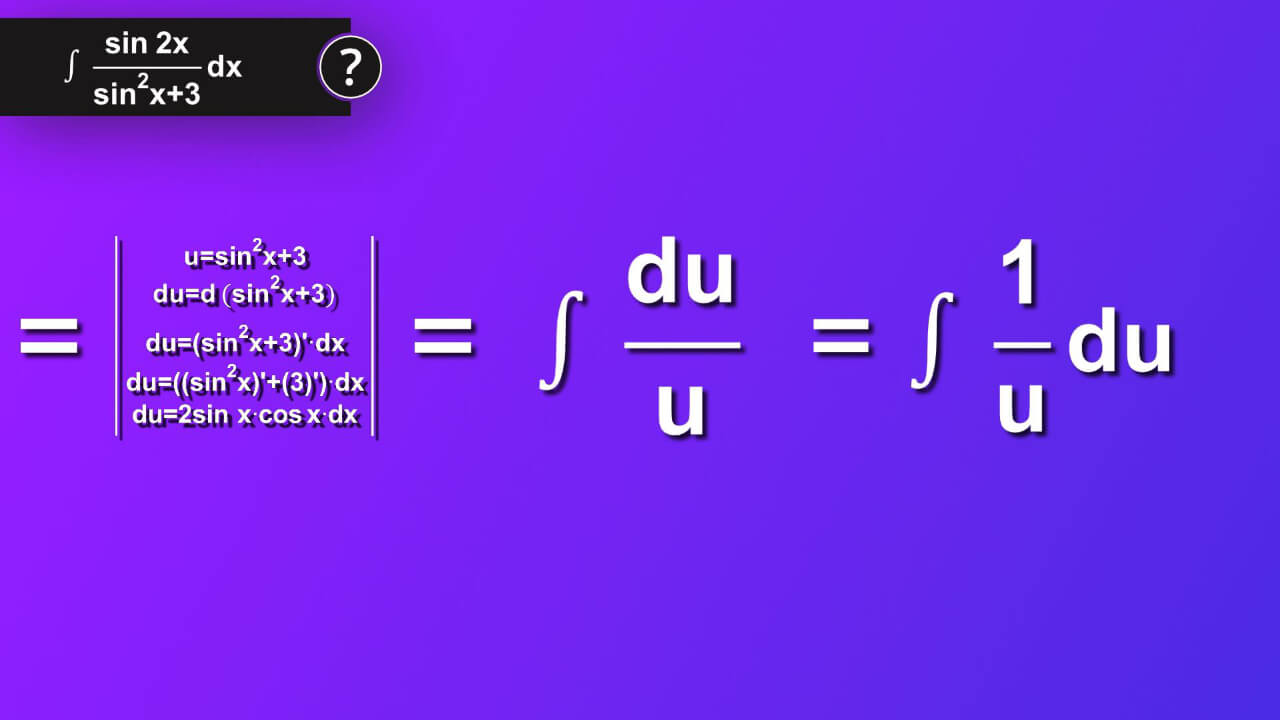### Step 6

Perfect, now we can apply formula for solving integral of one divided by s by ds which equals natural logarithm of absolute value of s. We also must add constant C to make sure that we have all our solution taken into account as our solution make whole class of function not a single one. In our case s is equal to u.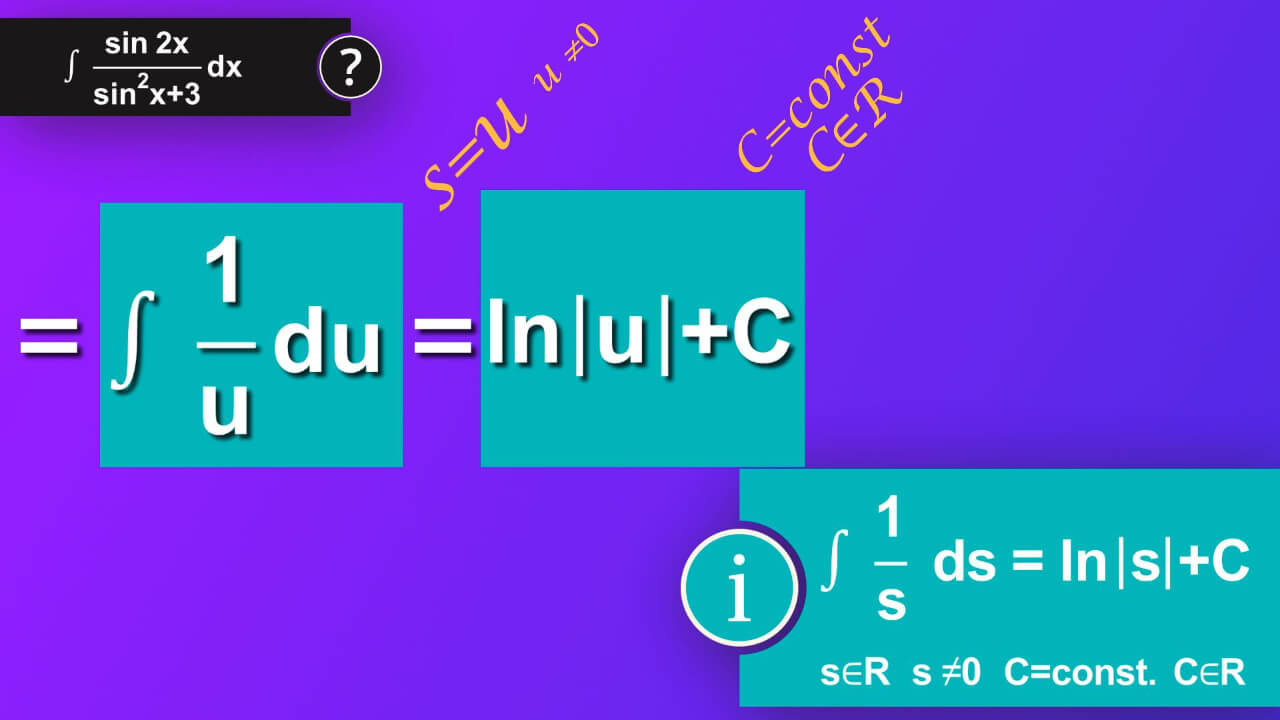### Step 7

Then, we’ve got natural logarithm of absolute value of u plus constant C. But it is not the end of our solution. We must come back to our problem, and it was formulated using variable x. We used u-substitution and now we must come back to x. So instead of u we now insert square sine of x plus 3.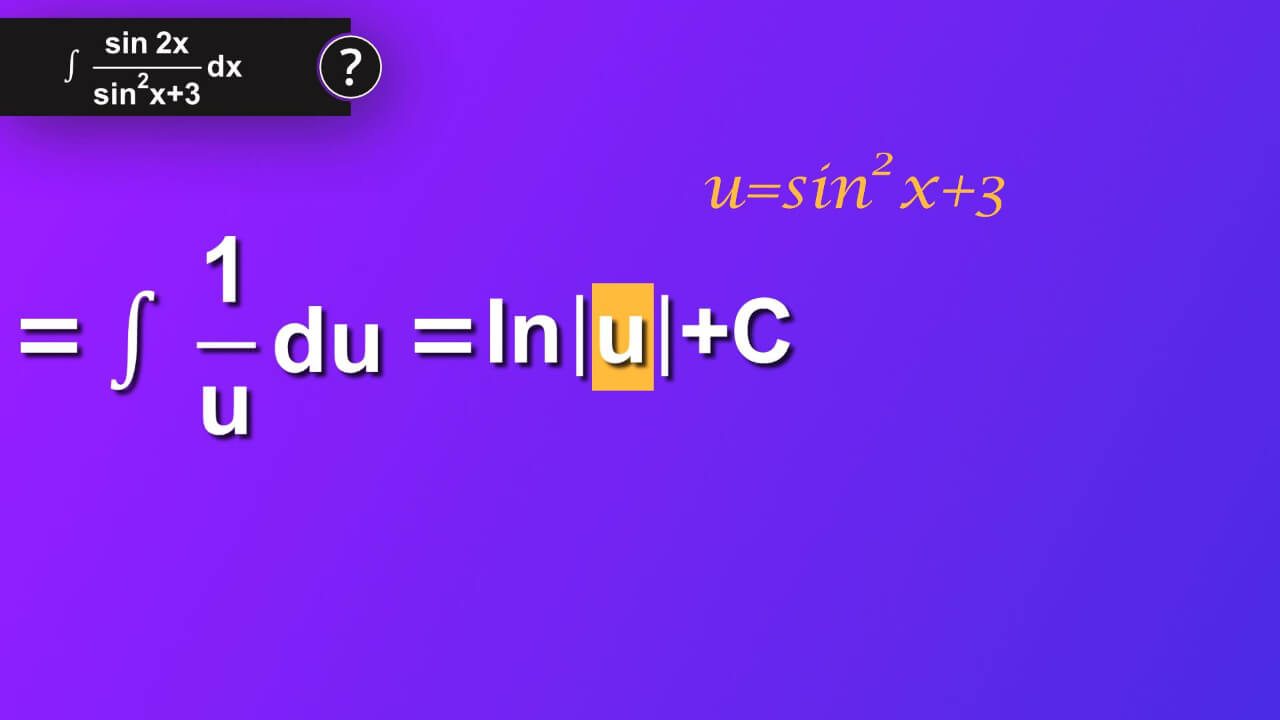### Step 8

We’ve got natural logarithm of absolute value of ( square sine of x plus 3) plus constant C as a result of our problem.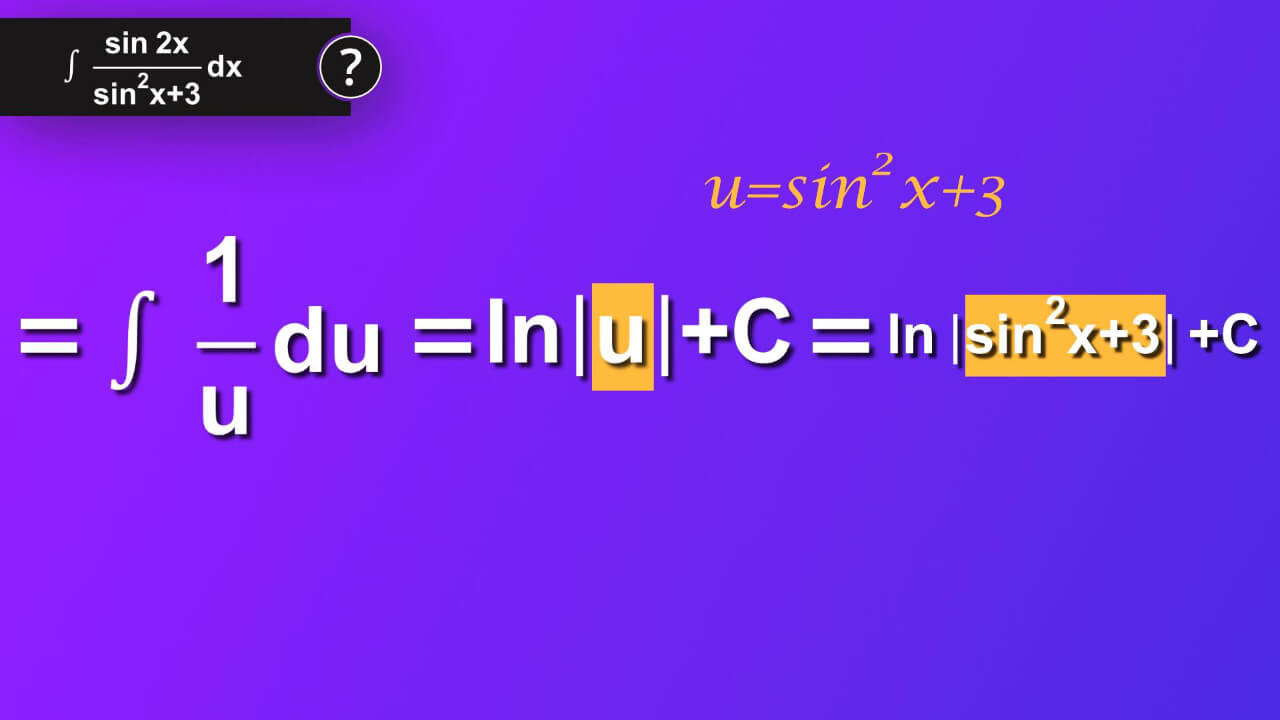## What is indefinite integral of sine 2x divided by square sine of x plus 3 by dx?

We finally did it: ∫ ((sin 2x)/(sin^2 x +3))dx=ln|(sin^2)x+3|+C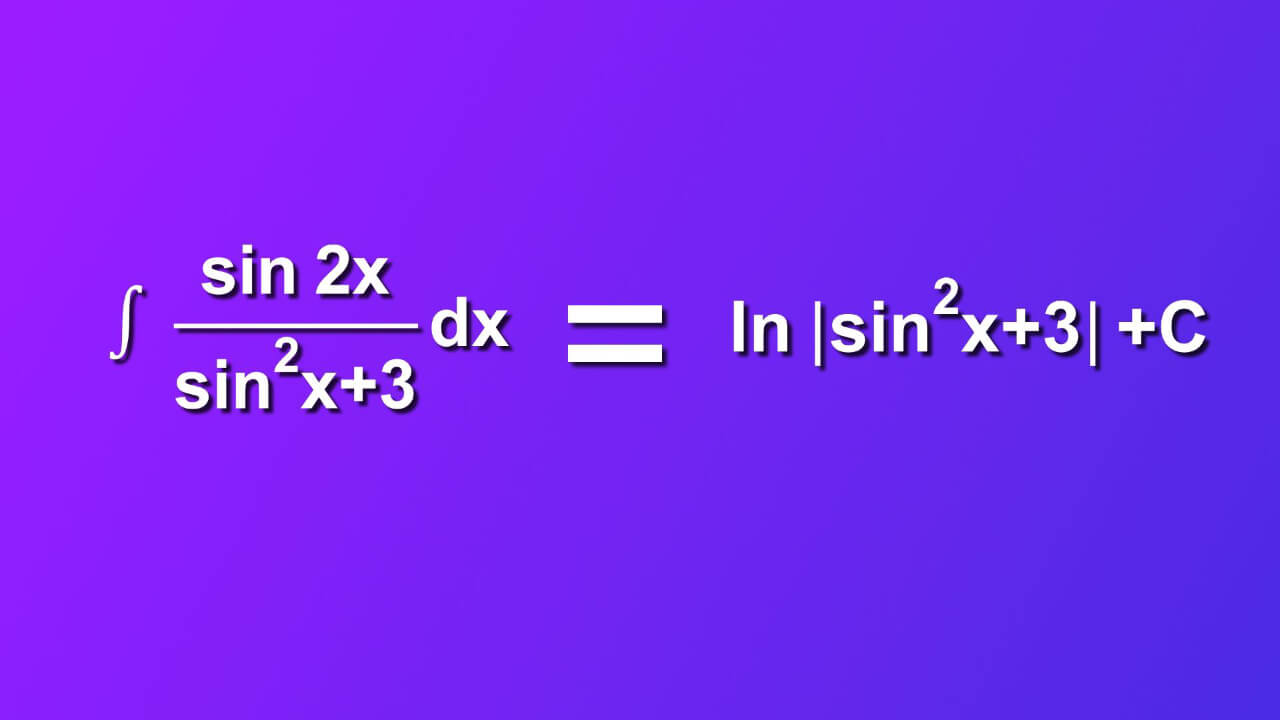## Dictionary

### Integration (antidifferentiation)

Computation (process of finding) of an integral, opposite process to differentiation.

### Integrand

Function placed between sign of integral and differential of variable of integration e.g. $${{ \int f(x)dx}}$$,
where:
$${{ \int }}$$- integration operator,
f(x) – integrand,
dx- differential of variable of integration x

### Integrable function

Function that integral over its domain is finite.

### Indefinite integral

Represents a class of primitive functions whose derivative is the integrand e.g. $${{\int f(x)dx=F(x)+C \Leftrightarrow F’(x)=f(x)}}$$
$${{C=const. }}$$,
$${{f, F,C \in R }}$$
R-real numbers
$${{\int f(x)dx}}$$ - indefinite integral of function f(x) by dx,
$${{F(x)+C}}$$ – a class of primitive functions that $${{F’(x)=f(x) }}$$,
F(x) - primitive function, usually written in capital letters,
R-real numbers.

### Integration by substitution, substitution method, u-substitution

Method used to solve integral when an integral contains some function and its derivative. The function we sat as equal to u and we rewrite the integral using a new variable u.
$${{\varphi: x \rightarrow \varphi (x)=u, x \in X, u \in U}}$$
and
$${{f: u \rightarrow f(u), u \in U, f(u) \in \varphi }}$$
$${{\varphi}}$$ is injective and differentiable function in X
f is continuous in U
then, $${{ \int f(\varphi (x)) \varphi’(x)dx=f(u) du}}$$ $${{\int f(\varphi (x))\varphi’(x)dx=\Bigg|\matrix{u= \varphi (x) \cr d(u)= d(\varphi (x))\cr du= \varphi ’(x) dx}\Bigg| =\int f(u) du}}$$

### Function

Function specified on a set X and having values in set Y is an assignment each element of set X specifically one element in set Y.
$${{f: X \rightarrow Y}}$$
f-function name,
X-set of elements of function f, domain of a function f
Y-set of function values of function f, codomain of a function f
$${{x\in X, y \in Y}}$$
$${{y=f(x) f: x\rightarrow y}}$$
$${{y=y(x), }}$$
y(x)-vales of the function named y,
x-independent variable,
y-dependent variable.

### Composition of functions

Function f(x) and g(x) are specified on set X,
$${{h: X\rightarrow T}}$$ and h(x)=t for x\inX and t\inT
$${{g: T\rightarrow Y}}$$
$${{x\in X, t\inT, y\in Y}}$$
$${{x \buildrel h\over\longrightarrow h(x) \buildrel g\over\longrightarrow g(t)=y}}$$
$${{x\rightarrow y=g(t)|_{t=h(x)}=g(h(x)) }}$$
$${{g(h(x)) }}$$ is a composition of two functions $${{ (g \circ h) (x) }}$$
$${{x \buildrel { g \circ h }\over\longrightarrow y=(g \circ h) (x) }}$$
where:
h-internal function,
g-external function.

### Derivative, derivative of a function

If $${{lim {\Delta f \over \Delta x }}}$$exists (and is finite), then this limit is named
$${{ \Delta x\rightarrow 0}}$$
derivative of a function f(x) in a point x0 and use symbol f’(x).
$${{ f’(x_0) {\buildrel\rm def\over=} \lim_{\Delta x\to0} {\Delta f \over \Delta x}}}$$

### Derivative function f’(x)

Derivative function of function f(x) is a function that values in a point x_0 are equal to derivative of this function in the point x_0.
f’(x_0) - derivative of a function f(x) in a point x_0 (a number, because it is a specifically determined limit of a function),
f’(x)-derivative function.

### Differentiable function

f(x) is differentiable in a point x_0 means that there exists f’(x_0 )
-f(x) is differentiable in (a,b) means there exists f’(x), x $${{\in(a,b) }}$$
-f(x) is differentiable in means there exists $${{f’(x), x \in}}$$

### Differential of a function (df)

Differential of a function f(x) in a point x_0 is symbolized as df(x_0) and $${{df(x_0) {\buildrel\rm def\over=} f’(x_0) \Delta x}}$$